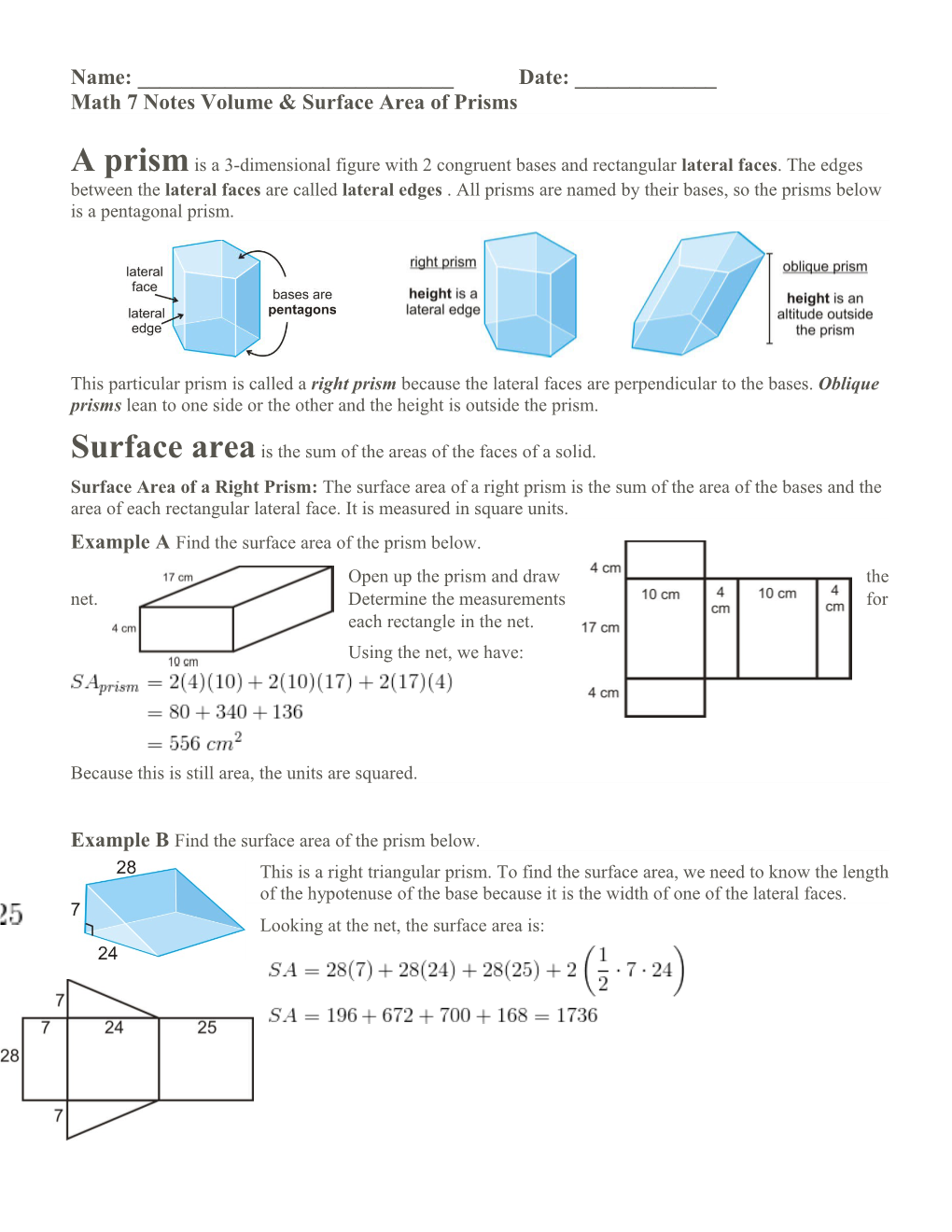# Math 7 Notes Volume & Surface Area of PrismsName: ______Date: ______

Math 7 Notes Volume & Surface Area of Prisms

A prism is a 3-dimensional figure with 2 congruent bases and rectangular lateral faces. The edges between the lateral faces are called lateral edges . All prisms are named by their bases, so the prisms below is a pentagonal prism.

This particular prism is called a right prism because the lateral faces are perpendicular to the bases. Oblique prisms lean to one side or the other and the height is outside the prism.

Surface areais the sum of the areas of the faces of a solid.

Surface Area of a Right Prism: The surface area of a right prism is the sum of the area of the bases and the area of each rectangular lateral face. It is measured in square units.

Example AFind the surface area of the prism below.

Open up the prism and draw the net. Determine the measurements for each rectangle in the net.

Using the net, we have:

Because this is still area, the units are squared.

Example B Find the surface area of the prism below.

This is a right triangular prism. To find the surface area, we need to know the length of the hypotenuse of the base because it is the width of one of the lateral faces.

Looking at the net, the surface area is:

Volumeis the measure of how much space a three-dimensional figure occupies. The basic unit of volume is the cubic unit: cubic centimeter , cubic inch , cubic meter , cubic foot , etc. Each basic cubic unit has a measure of one for each: length, width, and height.

Volume of a Rectangular Prism: If a rectangular prism is units high, units wide, and units long, then its volume is . If we further analyze the formula for the volume of a rectangular prism, we would see that is equal to the area of the base of the prism, B, a rectangle. If the bases are not rectangles, this would still be true, however we would have to rewrite the equation a little.

Volume of a Prism: If the area of the base of a prism is and the height is , then the volume, .

Cavalieri’s Principle: If two solids have the same height and the same cross-sectional area at every level, then they will have the same volume.

Basically, if an oblique prism and a right prism have the same base area and height, then they will have the same volume.

Example C A typical shoe box is 8 in by 14 in by 6 in. What is the volume of the box?

We can assume that a shoe box is a rectangular prism. Therefore, we can use the formula above.

Example D You have a small, triangular prism shaped tent. How much volume does it have, once it is up?

First, we need to find the area of the base. That is going to be . Multiplying this by 7 we would get the entire volume. The volume is .

Even though the height in this problem does not look like a “height,” it is, when referencing the formula. Usually, the height of a prism is going to be the last length you need to use.

Example E What if your family were ready to fill the new pool with water and they didn't know how much water would be needed? The shallow end is 4 ft. and the deep end is 8 ft. The pool is 10 ft. wide by 25 ft. long. How many gallons of water will it take to fill the pool? There are approximately 7.48 gallons in a cubic foot.

Even though it doesn’t look like it, the trapezoid is considered the base of this prism. The area of the trapezoidsare . Multiply this by the height, 10 ft, and we have that the volume is . To determine the number of gallons that are needed, divide 1500 by 7.48. gallons are needed to fill the pool.

Watch this video for help with the Examples above. CK-12 Foundation: Chapter11PrismsB

Name: ______Date: ______

Math 7 Prep Task Volume & Surface Area of Prisms

1. Find the volume of the right rectangular prism below.

Use the right triangular prism to answer questions 2-6.

2. What shape are the bases of this prism? What are their areas?

3. What are the dimensions of each of the lateral faces? What are their areas?

4. Find the lateral surface area of the prism.

5. Find the total surface area of the prism.

6. Find the total volume of the prism.

7. Writing Describe the difference between lateral surface area and total surface area.

______

______

8. Fuzzy dice are cubes with 4 inch sides.

a. What is the surface area of one die?

b. Typically, the dice are sold in pairs. What is the surface area of two dice?

c. What is the volume of both dice?

9. Find the surface area and volume of the following solid. Bases are isosceles trapezoids

EXTRA EXAMPLES

Find the value of , given the surface area.

Use the diagram below for questions 13-16. The barn is shaped like a pentagonal prism with dimensions shown in feet.

1. What is the area of the roof? (Both sides)
2. What is the floor area of the barn?
3. What is the area of the sides of the barn?
4. What is the total volume of the barn?